MATHEMATICS

 Time: Two Hours (8.30 AM – 10.30 AM) Max.Marks: 50

NOTE:-

1.      Attempt all questions.

2.      Rough work must be enclosed with answer book.

3.      While answering, refer to a question by its serial number as well as section heading. (eg.Q2/Sec.A)

4.      There is no negative marking.

5.      Answer each of Sections A, B, C at one place.

6.      Elegant solutions will be rewarded.

7.      Use of calculators, slide rule, graph paper and logarithmic, trigonometric and statistical tables is not permitted.

Note:-  All answers to questions in Section-A, Section-B and Section-C  must be supported by mathematical arguments.  In each of these sections order of the questions must be maintained.

SECTION-A

This section has Five Questions.  Each question is provided with five alternative answers.  Only one of them is the correct answer.  Indicate the correct answer by A, B, C, D, E.

(5x2=10 MARKS)

1.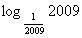=

A)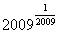B) -2009                   C)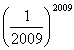D) -1                        E) 1

2.   Let a, b, c be the lengths of the sides of a triangle ABC.  Let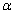,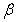,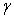be positive integers and     p =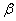a+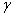b+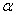c, q =a+b+c and r=a+b+c.Then p, q, r are lengths of the sides of a triangle

A) only if,,are all distinct           B) only if+>,+>,+>C) only if>>D) only if>a,>b,>c

E) for all values of,,3.   Let f:R à R be defined by f(x) = x2 for all x in R.  Let A = {4, 3, 0, -1}.  Then

A) f -1(A) is not defined as f -1 does not exist

B) For any function f: PàQ, if T is a subset of Q, then f -1(T) is defined and here  f -1(A) = {-2, 2,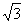, -, 0}

C) f -1(A) is not defined as ‘-1’ has no pre-image under the function f

D) f -1(A) = f (A)                                                        E) None of these

4.   If 1216451*0408832000 is equal to 19!, where * denotes a digit, then the missing digit represented by * is

A) 0                          B) 4                          C) 2                          D) 5                          E) 7

5.   A polynomial f(x) is said to be reducible if it can be written as f(x) = g(x)h(x) where        deg(g(x)) > 1 and deg(h(x))>1.  Otherwise it is said to be irreducible.

If f(x) is a polynomial of degree 2009,  Choose the correct statement from the following.

A) There are infinitely many f(x) that are irreducible

B) There are exactly 2009 f(x) that are irreducible    C) f(x)=x2009 +1 is an irreducible polynomial

D) There exists no f(x) that is irreducible                   E) none of these

SECTION-B

This section has Five Questions.  In each question a blank is left.  Fill in the blank.

(5x2=10 MARKS)

1.   The number of positive integers less than 2009 that have exactly three prime factors, not necessarily distinct, such that each prime factor is greater than 10 is _____________

 2.  If the odd natural numbers are arranged as shown in the figure, then the extremities of the row containing the number 2009 are _____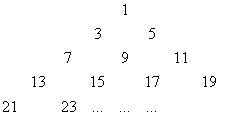3.   The number of triangles having a fixed base of ‘a’ units and area 2009 square units is _________

4.   The coefficient of x in the expansion of (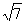x4 +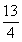x3 + x2 + 2x – 1)2009 is ___________

 5.   There are four circles in the figure.  Two of them have radius 2 and the smallest one has radius 1.  The radius of the largest circle, if the area of the dashed part is equal to the area of the dotted part, is __________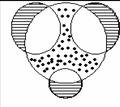SECTION-C                                (5x2=10 MARKS)

1.   How many positive integers less than 2009 are divisible by any one perfect square greater than 1678?

 2.   The square ABCD of side ‘a’ and the square BEFG of side ‘b’ are drawn next to each other as shown in the figure.  Express in terms of a and b the area of the quadrilateral formed by the mid points of the line segments AB, BE, FC and DG.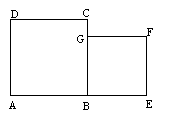3.   Find out the unequal pairs of sets, if any, among the following sets.

i)   (A-B)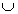(B-A)                         ii) (A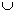B)-(A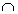B)                              iii) (AB)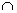(AB)’

iv) (AB’)(BA’)                 v) (AB)(A’B’)

4.   If ABC is a triangle with AB, BC measuring p and q units respectively, find the maximum area possible for triangle ABC.

5.   Two real numbers x and y are varying such that their sum is 2009.  Find the values of x and y such that their product is maximum.

SECTION-D                                (5x4=20 MARKS)

1.   Solve 2x2y – 3x2 + 4y = 2009 for x and y in positive integers.

 2.   The lower part of the house (see the Figure) in the circle is a square, and the top is an equilateral triangle. Find a relation between the length of the side of the house and  the radius of the circle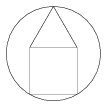3.   A function f: NàZ  is defined by f(n) =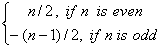Is f a bijection?

4.   Solve the system of equations ax + 2y = b and 2x – y= 3 for all real values of a and b.

5.   Is it possible to insert exactly 125 Harmonic Means between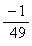and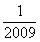? If so, what is the 6th HM?